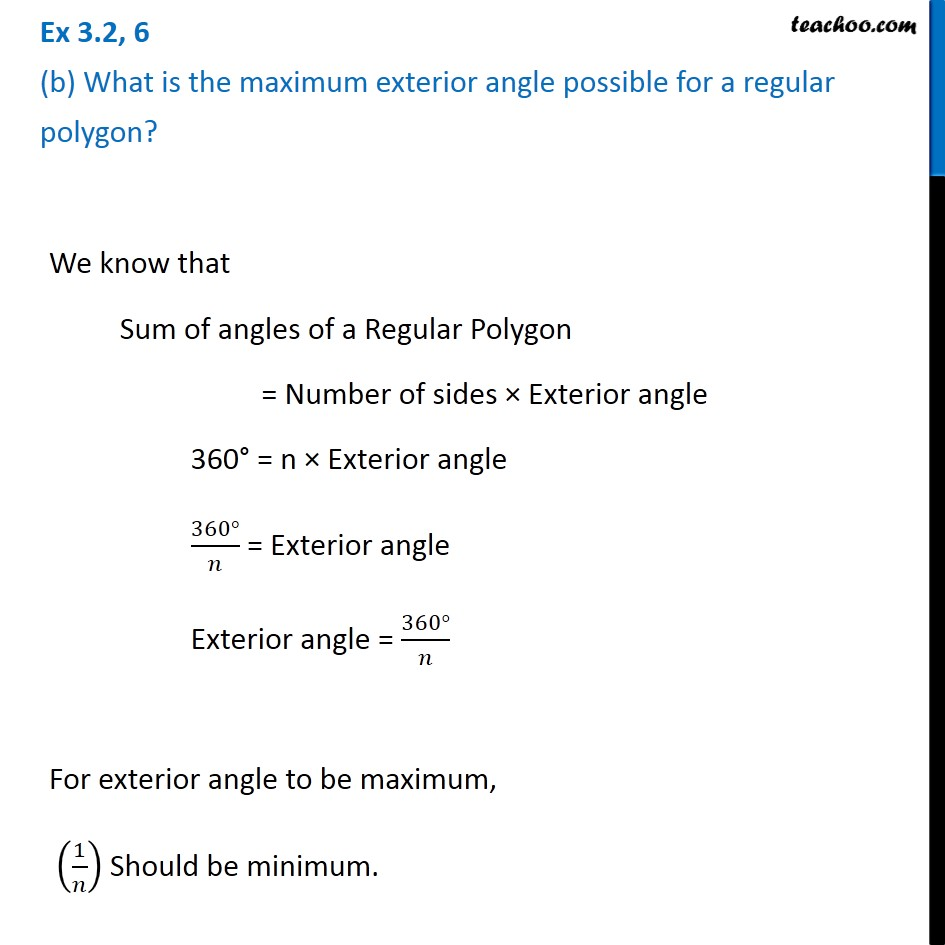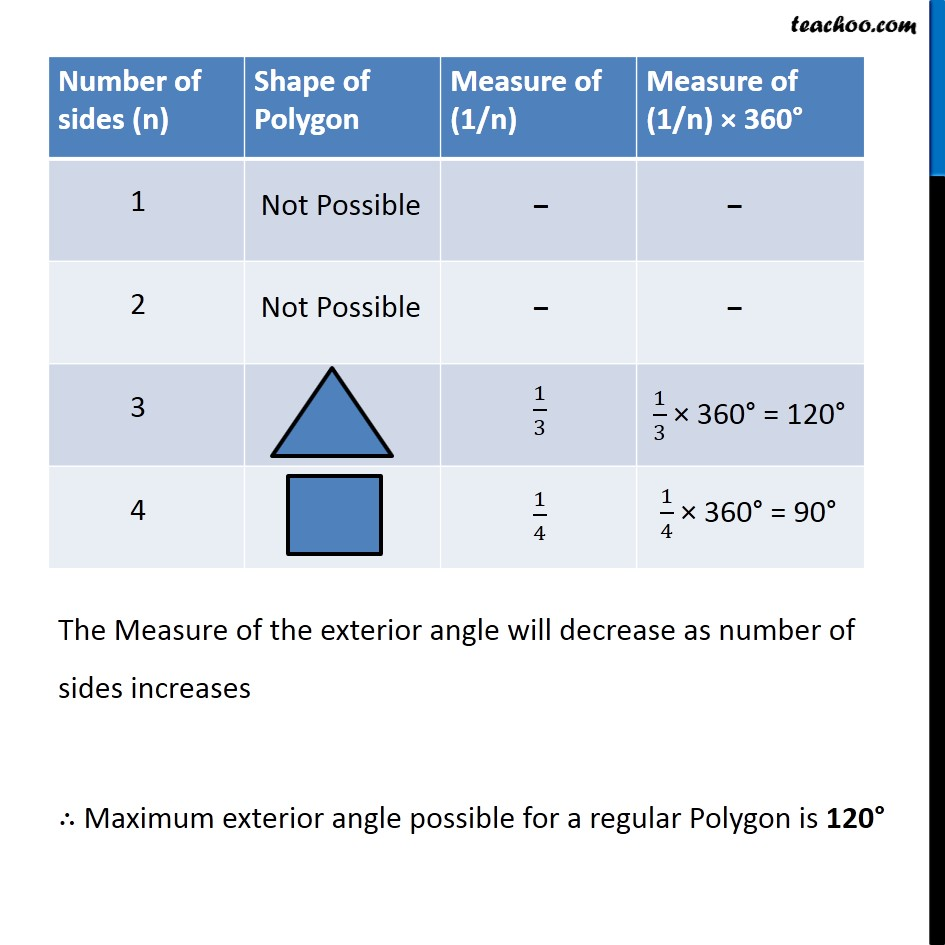Ex 3.2

Chapter 3 Class 8 Understanding Quadrilaterals
Serial order wiseLearn in your speed, with individual attention - Teachoo Maths 1-on-1 Class

### Transcript

Ex 3.2, 6 (b) What is the maximum exterior angle possible for a regular polygon? We know that Sum of angles of a Regular Polygon = Number of sides × Exterior angle 360° = n × Exterior angle (360°)/𝑛 = Exterior angle Exterior angle = (360°)/𝑛 For exterior angle to be maximum, (1/𝑛) Should be minimum. The Measure of the exterior angle will decrease as number of sides increases ∴ Maximum exterior angle possible for a regular Polygon is 120°